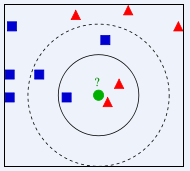# 用Node.js实现机器学习中的K最近邻分类算法

## 1. 简介

### k-nearest-neighbor-classifier

balabala了这么多，是不是某些同学想大喊一声..speak Chinese! 还是来通俗的解释下，然后再来看上面的理论应该会明白很多。小时候妈妈会指着各种各样的东西教我们，这是小鸭子，这个红的是苹果等等，那我们哼哧哼哧的看着应答着，多次被教后再看到的时候我们自己就能认出来这些事物了。主要是因为我们在脑海像给这个苹果贴了很多标签一样，不只是颜色这一个标签，可能还有苹果的形状大小等等。这些标签让我们看到苹果的时候不会误认为是橘子。其实这些标签就对应于机器学习中的特征这一重要概念，而训练我们识别的过程就对应于泛化这一概念。一台iphone戴了一个壳或者屏幕上有一道划痕，我们还是能认得出来它，这对于我们人来说非常简单，但蠢计算机就不知道怎么做了，需要我们好好调教它，当然也不能过度调教2333，过度调教它要把其他手机也认成iphone那就不好了，其实这就叫过度泛化。## 2. 实现

``````    // node.js用来读取xls文件的包
var xls = require('node-xlrd');
``````

``````var data = [];
// 将文件中的数据映射到样本的属性
var map = ['a','b','c','d','e','f','g','h','i','j','k'];
// 读取文件
xls.open('data.xls', function(err,bk){
if(err) {console.log(err.name, err.message); return;}
var shtCount = bk.sheet.count;
for(var sIdx = 0; sIdx < shtCount; sIdx++ ){
var sht = bk.sheets[sIdx],
rCount = sht.row.count,
cCount = sht.column.count;
for(var rIdx = 0; rIdx < rCount; rIdx++){
var item = {};
for(var cIdx = 0; cIdx < cCount; cIdx++){
item[map[cIdx]] = sht.cell(rIdx,cIdx);
}
data.push(item);
}
}
// 等文件读取完毕后 执行测试
run();
});``````

``````
// Sample表示一个样本
var Sample = function (object) {
// 把传过来的对象上的属性克隆到新创建的样本上
for (var key in object)
{
// 检验属性是否属于对象自身
if (object.hasOwnProperty(key)) {
this[key] = object[key];
}
}
}``````

``````// SampleSet管理所有样本 参数k表示KNN中的k
var SampleSet = function(k) {
this.samples = [];
this.k = k;
};

// 将样本加入样本数组
this.samples.push(sample);
}``````

``````// 计算样本间距离 采用欧式距离
Sample.prototype.measureDistances = function(a, b, c, d, e, f, g, h, i, j, k) {
for (var i in this.neighbors)
{
var neighbor = this.neighbors[i];
var a = neighbor.a - this.a;
var b = neighbor.b - this.b;
var c = neighbor.c - this.c;
var d = neighbor.d - this.d;
var e = neighbor.e - this.e;
var f = neighbor.f - this.f;
var g = neighbor.g - this.g;
var h = neighbor.h - this.h;
var i = neighbor.i - this.i;
var j = neighbor.j - this.j;
var k = neighbor.k - this.k;

// 计算欧式距离
neighbor.distance = Math.sqrt(a*a + b*b + c*c + d*d + e*e + f*f + g*g + h*h + i*i + j*j + k*k);
}
};

// 将邻居样本根据与预测样本间距离排序
Sample.prototype.sortByDistance = function() {
this.neighbors.sort(function (a, b) {
return a.distance - b.distance;
});
};

// 判断被预测样本类别
Sample.prototype.guessType = function(k) {
// 有两种类别 1和-1
var types = { '1': 0, '-1': 0 };
// 根据k值截取邻居里面前k个
for (var i in this.neighbors.slice(0, k))
{
var neighbor = this.neighbors[i];
types[neighbor.trueType] += 1;
}

// 判断邻居里哪个样本类型多
if(types['1']>types['-1']){
this.type = '1';
} else {
this.type = '-1';
}
}``````

``````// 构建总样本数组，包含未知类型样本
SampleSet.prototype.determineUnknown = function() {
/*
* 一旦发现某个未知类型样本，就把所有已知的样本
* 克隆出来作为该未知样本的邻居序列。
* 之所以这样做是因为我们需要计算该未知样本和所有已知样本的距离。
*/
for (var i in this.samples)
{
// 如果发现没有类型的样本
if ( ! this.samples[i].type)
{
// 初始化未知样本的邻居
this.samples[i].neighbors = [];

// 生成邻居集
for (var j in this.samples)
{
// 如果碰到未知样本 跳过
if ( ! this.samples[j].type)
continue;
this.samples[i].neighbors.push( new Sample(this.samples[j]) );
}

// 计算所有邻居与预测样本的距离
this.samples[i].measureDistances(this.a, this.b, this.c, this.d, this.e, this.f, this.g, this.h, this.k);

// 把所有邻居按距离排序
this.samples[i].sortByDistance();

// 猜测预测样本类型
this.samples[i].guessType(this.k);
}
}
};``````

K倍交叉验证将所有样本分成K份，一般均分。取一份作为测试样本，剩余K-1份作为训练样本。这个过程重复K次，最后的平均测试结果可以衡量模型的性能。
k倍验证时定义了个方法先把数组打乱随机摆放。

``````// helper函数 将数组里的元素随机摆放
function ruffle(array) {
array.sort(function (a, b) {
return Math.random() - 0.5;
})
}``````

## 3. 总结

knn算法非常简单，但却能在很多关键的地方发挥作用并且效果非常好。缺点就是进行分类时要扫描所有训练样本得到距离，训练集大的话会很慢。看似高大上的机器学习其实就是基于计算机科学，统计学，数学的一些实现，相信通过这个最简单的入门算法能让我们对机器学习有一点小小的感知和体会。